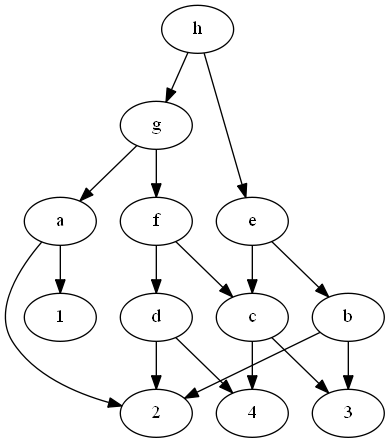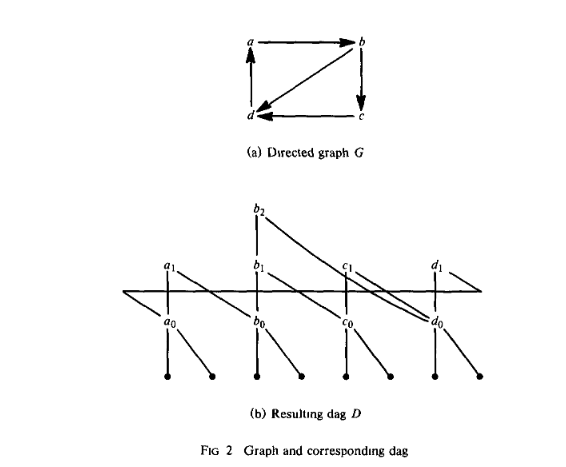# Monthly Archives: October 2021

## Code Generation on a One-Register Machine

I’ve done a lot of traveling over the past month, so I’m sorry about missing posts.  Things should be back to normal now.

The problem: Code Generation on a One-Register Machine.  This is problem PO4 in the appendix.

The description: Given a directed acyclic graph G = (V,A), in which no vertex has an out-degree larger than 2, and a positive integer K.  The leaves of this graph (the vertices with out-degree 0) are our starting values, sitting in memory.  Can we compute the values in all root vertices  (with in-degree 0) in K instructions or less, if our only instructions are:

• Load a value from memory into our only register.
• Store a value from the register into memory
• Doing an operation combining a value in a register and a value in memory.  The operation must connect two children of a vertex in a graph together, and the “result” is the parent vertex.  The result value replaces the original value in the register.

Example:  Here’s a simple graph:Here, we can compute the “+” node by:

• Doing the + operation between the register and 2
• Storing the + operation to memory (G&J’s definition of the problem says that the node is not computed until the value is stored)

We can compute the “-” node in another 3 instructions, and since the value of the “-‘ node is still in the register, compute the “*” node in 1 more instruction, and store it with out last instruction.

Here’s a more complicated graph:To do this one, we will have to load the value in node 2 lots of times.  For example, here is the set of instructions I came up with to compute h:

• op to create a (only 1 operand)
• store a
• op to create c (3 is in memory)
• store c
• op to create d (4 is in memory)
• op to create f (c is in memory)
• op to create g (a is in memory)
• store g
• op to create b (3 is in memory)
•  op to create e (c is in memory)
• op to create h (g is in memory)
• store h

It’s possible that we can do this in fewer instructions, but hopefully, you can see why this problem is hard- knowing what value to keep in the register is tricky.

Reduction: G&J point out that the reduction is from a paper by Bruno and Sethi, which uses 3SAT to do the reduction.  The instance they build is pretty complicated.  I also came across a paper by Aho, Johnson, and Ullman, who extend the result of the Bruno and Sethi paper with a nice reduction from Feedback Vertex Set.  I think this reduction is easier to follow, so we’ll go with that.

So, we are given an instance of FVS- a directed acyclic graph G and an integer K.  We are looking for a set F of K vertices such that every cycle goes in G goes through some element of F.

We are going to build our Code Generation graph D as follows:

• For every vertex in G with outdegree d, build a “left chain” of d+1 vertices.  So if vertex a had 2 vertices leaving it, we will create 3 vertices b0, b1, and b2.  b2 will connect to b1, and b1 will connect to b0.
• Each of the “0” vertices at the bottom of these chains connects to 2 distinct memory values (they will be the leaves of the code graph)
• If vertex v has outdegree d, each vertex in a’s chain will connect to the different “0” vertex of the different neighbors of v in G.

Here is an example from the paper:Notice that if we don’t have the edges between the chains, we can compute the entire chain with just 2 loads (of the leaves that start in memory).  So, the only loads needed to compute all of D happen in the leaves, or in some of the “level 1” vertices that are parents of the leaves.   If we have to re-load one of those vertices, it is because there is no optimal strategy to avoid loading it, which means it’s part of a cycle.

For example, look at the a and b chains in the picture above.  If we didn’t have any of the c or d vertices or edges in our graph, we could compute a1 and b1 without loading any vertex that is not a leaf: compute b0, b1, b2, then a0, then a1 (which uses a0 from the register and b0 from memory).  The reason we can do this is that while a1 depends on b0, none of the b vertices depend on anything in a, which gives us a chain to do first.  We need to reload a value when we have a circular dependency between chains (so there is no correct chain to do first).  That’s the relationship between the chains and the feedback vertex set.

This works in the other direction as well- if we are given the feedback vertex set in G, we can compute those vertices first in D, and then load them all once as needed to compute D.

The paper says that in the example graph, the node {d} by itself is a Feedback Vertex Set, and the optimal computation ordering is: d0,c0, c1, b0,b1, b2, a0,a1, d1.  That final d1 needs a re-load of d0.  The 1 extra load corresponds to the 1 vertex in our Feedback Set.

Difficulty: 6.  Maybe 7.  I think this is right at the limit of what a student can figure out, but I would also want a more rigorous proof about the connection between the extra loads and the feedback set, which is probably tricky to come up with.